Next: Momentum Representation Up: Fundamentals of Quantum Mechanics Previous: Ehrenfest's Theorem

# Operators

An operator,(say), is a mathematical entity which transforms one function into another: i.e.,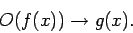(184)

For instance,is an operator, since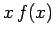is a different function to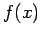, and is fully specified onceis given. Furthermore,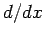is also an operator, since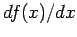is a different function to, and is fully specified onceis given. Now,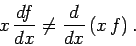(185)

This can also be written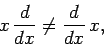(186)

where the operators are assumed to act on everything to their right, and a finalis understood [whereis a general function]. The above expression illustrates an important point: i.e., in general, operators do not commute. Of course, some operators do commute: e.g.,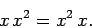(187)

Finally, an operator,, is termed linear if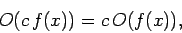(188)

whereis a general function, and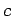a general complex number. All of the operators employed in quantum mechanics are linear.

Now, from Eqs. (158) and (174),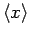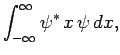(189)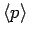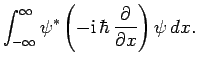(190)

These expressions suggest a number of things. First, classical dynamical variables, such asand, are represented in quantum mechanics by linear operators which act on the wavefunction. Second, displacement is represented by the algebraic operator, and momentum by the differential operator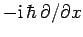: i.e.,(191)

Finally, the expectation value of some dynamical variable represented by the operator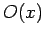is simply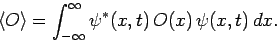(192)

Clearly, if an operator is to represent a dynamical variable which has physical significance then its expectation value must be real. In other words, if the operatorrepresents a physical variable then we require that, or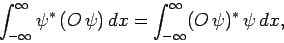(193)

whereis the complex conjugate of. An operator which satisfies the above constraint is called an Hermitian operator. It is easily demonstrated thatandare both Hermitian. The Hermitian conjugate,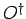, of a general operator,, is defined as follows: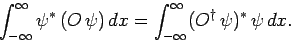(194)

The Hermitian conjugate of an Hermitian operator is the same as the operator itself: i.e.,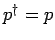. For a non-Hermitian operator,(say), it is easily demonstrated that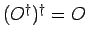, and that the operator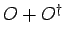is Hermitian. Finally, ifandare two operators, then.

Suppose that we wish to find the operator which corresponds to the classical dynamical variable. In classical mechanics, there is no difference betweenand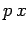. However, in quantum mechanics, we have already seen that. So, should be chooseor? Actually, neither of these combinations is Hermitian. However,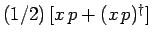is Hermitian. Moreover,, which neatly resolves our problem of which order to putand.

It is a reasonable guess that the operator corresponding to energy (which is called the Hamiltonian, and conventionally denoted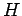) takes the form(195)

Note thatis Hermitian. Now, it follows from Eq. (191) that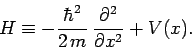(196)

However, according to Schrödinger's equation, (137), we have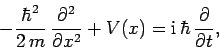(197)

so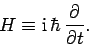(198)

Thus, the time-dependent Schrödinger equation can be written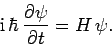(199)

Finally, ifis a classical dynamical variable which is a function of displacement, momentum, and energy, then a reasonable guess for the corresponding operator in quantum mechanics is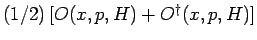, where, and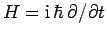.Next: Momentum Representation Up: Fundamentals of Quantum Mechanics Previous: Ehrenfest's Theorem
Richard Fitzpatrick 2010-07-20